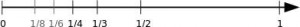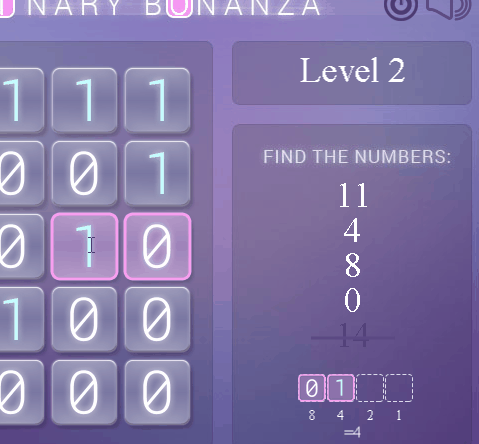## What are floating point errors? [Answered]

Computers are not always as accurate as we think. They do very well at what they are told to do and can do it very fast. But in many cases, a small inaccuracy can have dramatic consequences. A very well-known problem is floating point errors. Floating point numbers have limitations on how accurately a number can be represented. The actual number saved in memory is often rounded to the closest possible value. The accuracy is very high and out of scope for most applications, but even a tiny error can accumulate and cause problems in certain situations. Those situations have to be avoided through thorough testing in crucial applications. Many tragedies have happened – either because those tests were not thoroughly performed or certain conditions have been overlooked. [See: Famous number computing errors]

The following describes the rounding problem with floating point numbers.

## Errors in Floating Point Calculations

Every decimal integer (1, 10, 3462, 948503, etc.) can be exactly represented by a binary number. The only limitation is that a number type in programming usually has lower and higher bounds. For example, a 32-bit integer type can represent:

• 4,294,967,296 values in total
• Signed type: from -231 to 231-1 (-2,147,483,648 to 2,147,483,647 )
• Unsigned type: from 0 to 232-1 (0 to 4,294,967,295)

The limitations are simple, and the integer type can represent every whole number within those bounds.

However, floating point numbers have additional limitations in the fractional part of a number (everything after the decimal point). Again as in the integer format, the floating point number format used in computers is limited to a certain size (number of bits). As a result, this limits how precisely it can represent a number.

If the result of a calculation is rounded and used for additional calculations, the error caused by the rounding will distort any further results. Floating point numbers are limited in size, so they can theoretically only represent certain numbers. Everything that is inbetween has to be rounded to the closest possible number. This can cause (often very small) errors in a number that is stored. Systems that have to make a lot of calculations or systems that run for months or years without restarting carry the biggest risk for such errors.

Another issue that occurs with floating point numbers is the problem of scale. The exponent determines the scale of the number, which means it can either be used for very large numbers or for very small numbers. If two numbers of very different scale are used in a calculation (e.g. a very large number and a very small number), the small numbers might get lost because they do not fit into the scale of the larger number.

### Rounding in Decimal Numbers and Fractions

To better understand the problem of binary floating point rounding errors, examples from our well-known decimal system can be used. The fraction 1/3 looks very simple. Its result is a little more complicated: 0.333333333…with an infinitely repeating number of 3s. Even in our well-known decimal system, we reach such limitations where we have too many digits. We often shorten (round) numbers to a size that is convenient for us and fits our needs. For example, 1/3 could be written as 0.333.

What happens if we want to calculate (1/3) + (1/3)? If we add the results 0.333 + 0.333, we get 0.666. However, if we add the fractions (1/3) + (1/3) directly, we get 0.6666666. Again, with an infinite number of 6s, we would most likely round it to 0.667.

This example shows that if we are limited to a certain number of digits, we quickly loose accuracy. After only one addition, we already lost a part that may or may not be important (depending on our situation). If we imagine a computer system that can only represent three fractional digits, the example above shows that the use of rounded intermediate results could propagate and cause wrong end results.

To see this error in action, check out demonstration of floating point error (animated GIF) with Java code.

As in the above example, binary floating point formats can represent many more than three fractional digits. Even though the error is much smaller if the 100th or the 1000th fractional digit is cut off, it can have big impacts if results are processed further through long calculations or if results are used repeatedly to carry the error on and on.

### Binary Floating Point Numbers and Fractions

A very simple example:

When baking or cooking, you have a limited number of measuring cups and spoons available. You only have ¼, 1/3, ½, and 1 cup. So what can you do if 1/6 cup is needed? Or if 1/8 is needed? Those two amounts do not simply fit into the available cups you have on hand.

In real life, you could try to approximate 1/6 with just filling the 1/3 cup about half way, but in digital applications that does not work. Only the available values can be used and combined to reach a number that is as close as possible to what you need.Example of measuring cup size distribution.

The closest number to 1/6 would be ¼. It gets a little more difficult with 1/8 because it is in the middle of 0 and ¼. So one of those two has to be chosen – it could be either one. This gives an error of up to half of ¼ cup, which is also the maximal precision we can reach. The results we get can be up to 1/8 less or more than what we actually wanted.

Numbers in Computers

A computer has to do exactly what the example above shows. Since the binary system only provides certain numbers, it often has to try to get as close as possible. Naturally, the precision is much higher in floating point number types (it can represent much smaller values than the 1/4 cup shown in the example).

Binary integers use an exponent (20=1, 21=2, 22=4, 23=8, …), and binary fractional digits use an inverse exponent (2-1=½, 2-2=¼, 2-3=1/8, 2-4=1/16, …). For each additional fraction bit, the precision rises because a lower number can be used. With ½, only numbers like 1.5, 2, 2.5, 3, etc. are possible. With one more fraction bit, the precision is already ¼, which allows for twice as many numbers like 1.25, 1.5, 1.75, 2, etc.

A very common floating point format is the single-precision floating-point format. It has 32 bits and there are 23 fraction bits (plus one implied one, so 24 in total). [See: Binary numbers – floating point conversion] The smallest number for a single-precision floating point value is about 1.2*10-38, which means that its error could be half of that number.

For further details, do the following:Binary Bonanza Game